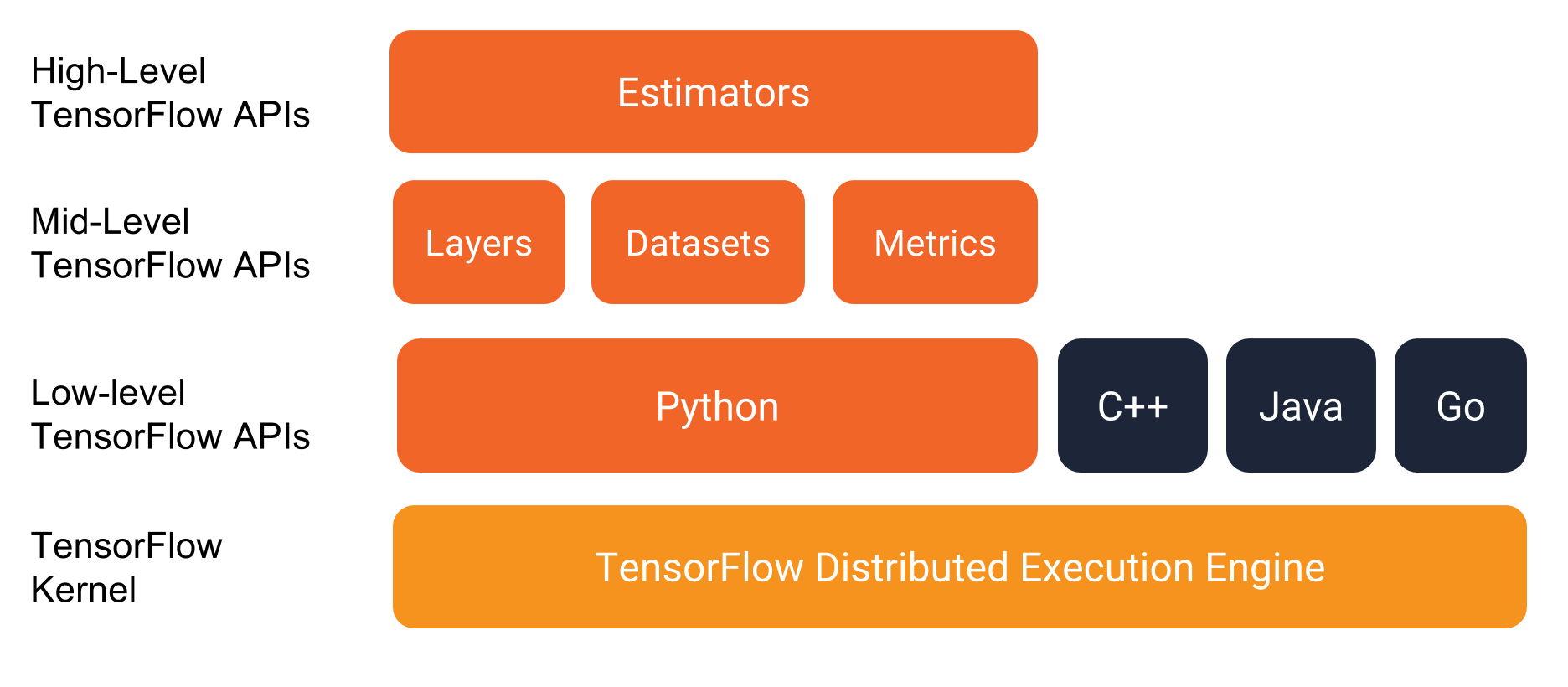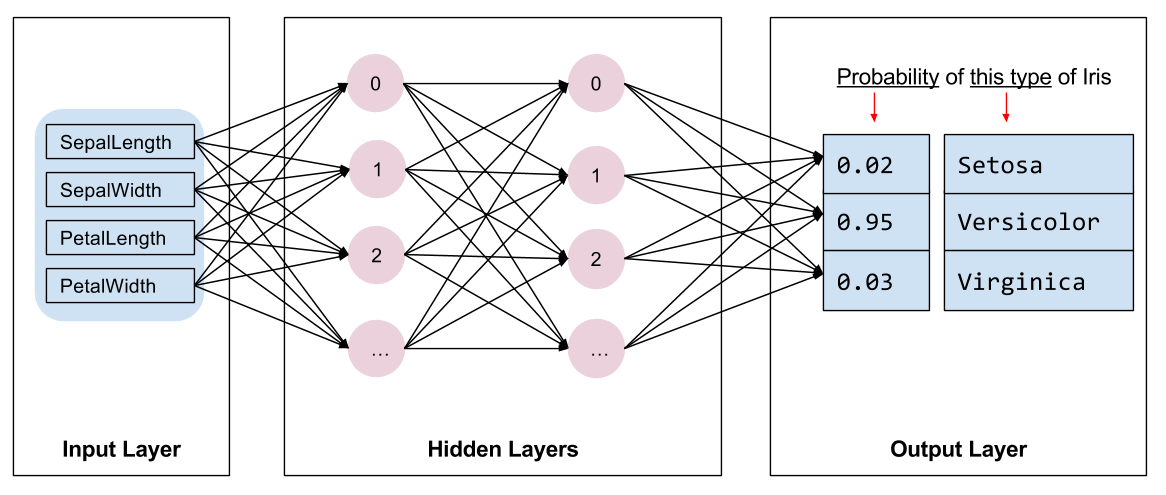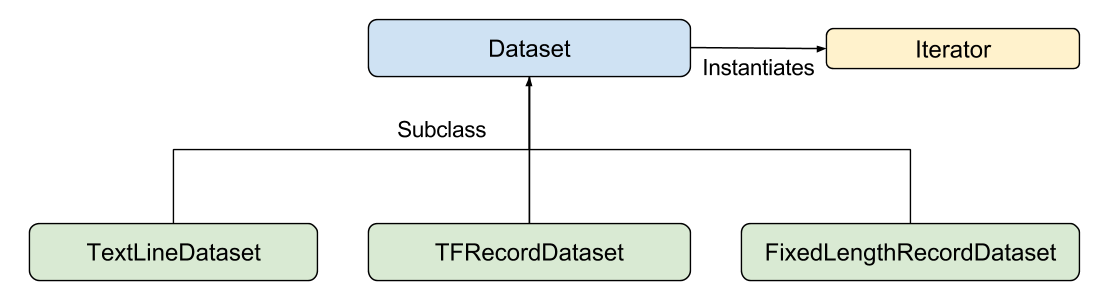## 前置要求

• 安装 TensorFlow
• 如果你在 virtualenv 或者 Anaconda 上安装了 TensorFlow，启动你的 TensorFlow 环境。
• 通过以下命令安装或升级 pandas：

``````  pip install pandas
``````

## 获取样例代码

1. 通过键入以下命令从 GitHub 下载 TensorFlow Models 仓库：

`````` git clone https://github.com/tensorflow/models
``````
2. 进入含有样例的文件夹：

`````` cd models/samples/core/get_started/
``````

`premade_estimator.py`.

`iris_data.py`

### 运行程序

``````python premade_estimator.py
``````

``````...
Prediction is "Setosa" (99.6%), expected "Setosa"

Prediction is "Versicolor" (99.8%), expected "Versicolor"

Prediction is "Virginica" (97.9%), expected "Virginica"
``````

• 你正确地安装 TensorFlow 了吗？
• 你使用的是正确版本的 TensorFlow 吗？
• 你启动安装了 TensorFlow 的环境了吗？（这条仅会出现在一些特定的安装方法中）

## 程序栈## 对　irises　分类：概览Iris setosa (by
Iris versicolor (by
Dlanglois, CC BY-SA 3.0),
and Iris virginica
(by Frank Mayfield, CC BY-SA
2.0).

### 数据集

Iris 数据集包含四个特征和一个标签。四个特征定义了 Iris 花的植物特征：

• 花萼长度
• 花萼宽度
• 花瓣长度
• 花瓣宽度

• Iris setosa (0)
• Iris versicolor (1)
• Iris virginica (2)

5.1 3.3 1.7 0.5 0 (Setosa)
5.0 2.3 3.3 1.0 1 (versicolor)
6.4 2.8 5.6 2.2 2 (virginica)

### 算法

• 2 个隐藏层。
• 每个隐藏层拥有 10 个节点。### 接口

• Iris Setosa 的可能性为 0.03
• Iris Versicolor 的可能性为 0.95
• Iris Virginica 的可能性为 0.02

## 使用 Estimators 编程的概览

`tf.estimator.Estimator`

• 创建一个或多个输入函数。
• 定义模型的特征列。
• 实例化一个 Estimator，定义特征列和各类超参数。
• 在 Estimator 对象上调用一个或多个方法，传入合适的输入函数来作为数据源。

## 创建输入函数

`tf.data.Dataset`

• `features` - 一个 Python 字典：
每个键为特征的名字。     每个值为一个包含所有该特征的值的数组。
• `label` - 一个数组包含着所有例子的标签

``````def input_evaluation_set():
features = {'SepalLength': np.array([6.4, 5.0]),
'SepalWidth':  np.array([2.8, 2.3]),
'PetalLength': np.array([5.6, 3.3]),
'PetalWidth':  np.array([2.2, 1.0])}
labels = np.array([2, 1])
return features, labels
``````• `Dataset` - 包含创建和传输数据集的基类。同时也允许你通过内存中的数据来初始化数据集，或从一个 Python 生成器。
• `TextLineDataset` - 从文本文件逐行读取
• `TFRecordDataset` - 从 TFRecord 文件读取记录
• `FixedLengthRecordDataset` - 从二进制文件读取固定大小的记录
• `Iterator` - 提供了一种每次获取数据集中的元素的方法

Dataset API 可以为你处理很多常见的情况。例如，使用它可以简单地从一系列巨大的文件中并行地读取记录并合成一个单个的流。

``````def train_input_fn(features, labels, batch_size):
"""训练中使用的输入函数"""
# 将输入变为一个 Dataset
dataset = tf.data.Dataset.from_tensor_slices((dict(features), labels))

# 打乱，重复并将样例分批次
return dataset.shuffle(1000).repeat().batch(batch_size)
``````

## 定义特征列

`tf.feature_column`

``````# Feature columns describe how to use the input.
my_feature_columns = []
for key in train_x.keys():
my_feature_columns.append(tf.feature_column.numeric_column(key=key))
``````

## 实例化一个 Estimator

Iris 问题是一个经典的分类问题。幸运的是，TensorFlow 提供了一些预制的 Estimator 分类器，包含：

``````# 创建一个有两个隐藏层和每层10个节点的 DNN
classifier = tf.estimator.DNNClassifier(
feature_columns=my_feature_columns,
# 两个隐藏层，每层 10 个节点。
hidden_units=[10, 10],
# 模型必须在 3 个类别中作出选择
n_classes=3)
``````

## 训练，评估和预测

• 训练模型
• 评估训练好的模型
• 使用训练好的模型进行预测

### 训练模型

``````# 训练模型
classifier.train(
input_fn=lambda:iris_data.train_input_fn(train_x, train_y, args.batch_size),
steps=args.train_steps)
``````

### 评估训练好的模型

``````# 评估该模型
eval_result = classifier.evaluate(
input_fn=lambda:iris_data.eval_input_fn(test_x, test_y, args.batch_size))

print('\nTest set accuracy: {accuracy:0.3f}\n'.format(**eval_result))
``````

``````Test set accuracy: 0.967
``````

### 通过训练好的模型进行预测

``````# 通过模型进行预测
expected = ['Setosa', 'Versicolor', 'Virginica']
predict_x = {
'SepalLength': [5.1, 5.9, 6.9],
'SepalWidth': [3.3, 3.0, 3.1],
'PetalLength': [1.7, 4.2, 5.4],
'PetalWidth': [0.5, 1.5, 2.1],
}

predictions = classifier.predict(
input_fn=lambda:iris_data.eval_input_fn(predict_x,
batch_size=args.batch_size))
``````

`predict` 方法返回了一个 Python 的可迭代类型，是一个含有每个例子的预测结果的字典。如下的代码打印出了一些预测和它们的可能性：

``````template = ('\nPrediction is "{}" ({:.1f}%), expected "{}"')

for pred_dict, expec in zip(predictions, expected):
class_id = pred_dict['class_ids']
probability = pred_dict['probabilities'][class_id]

print(template.format(iris_data.SPECIES[class_id],
100 * probability, expec))
``````

``````...
Prediction is "Setosa" (99.6%), expected "Setosa"

Prediction is "Versicolor" (99.8%), expected "Versicolor"

Prediction is "Virginica" (97.9%), expected "Virginica"
``````